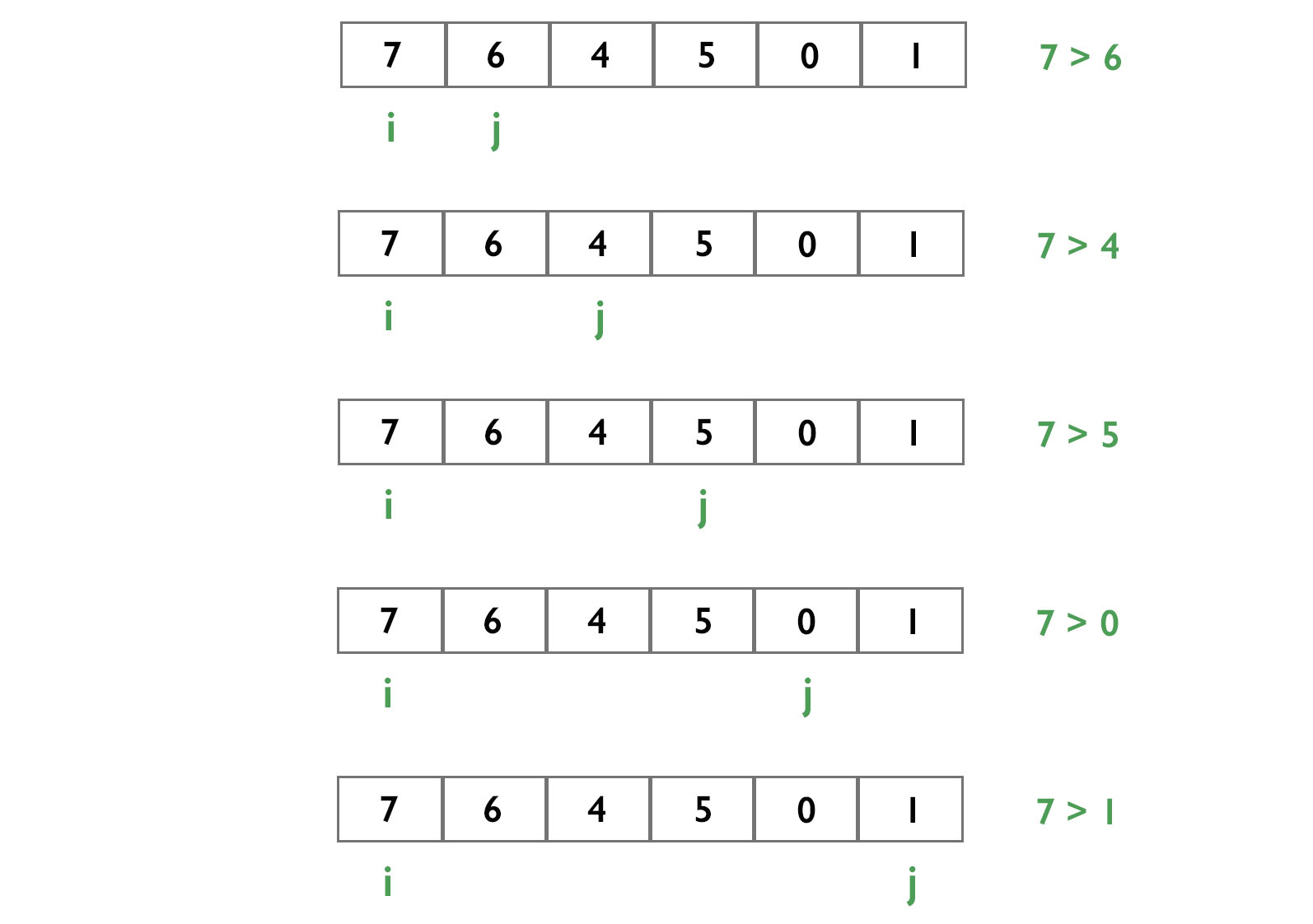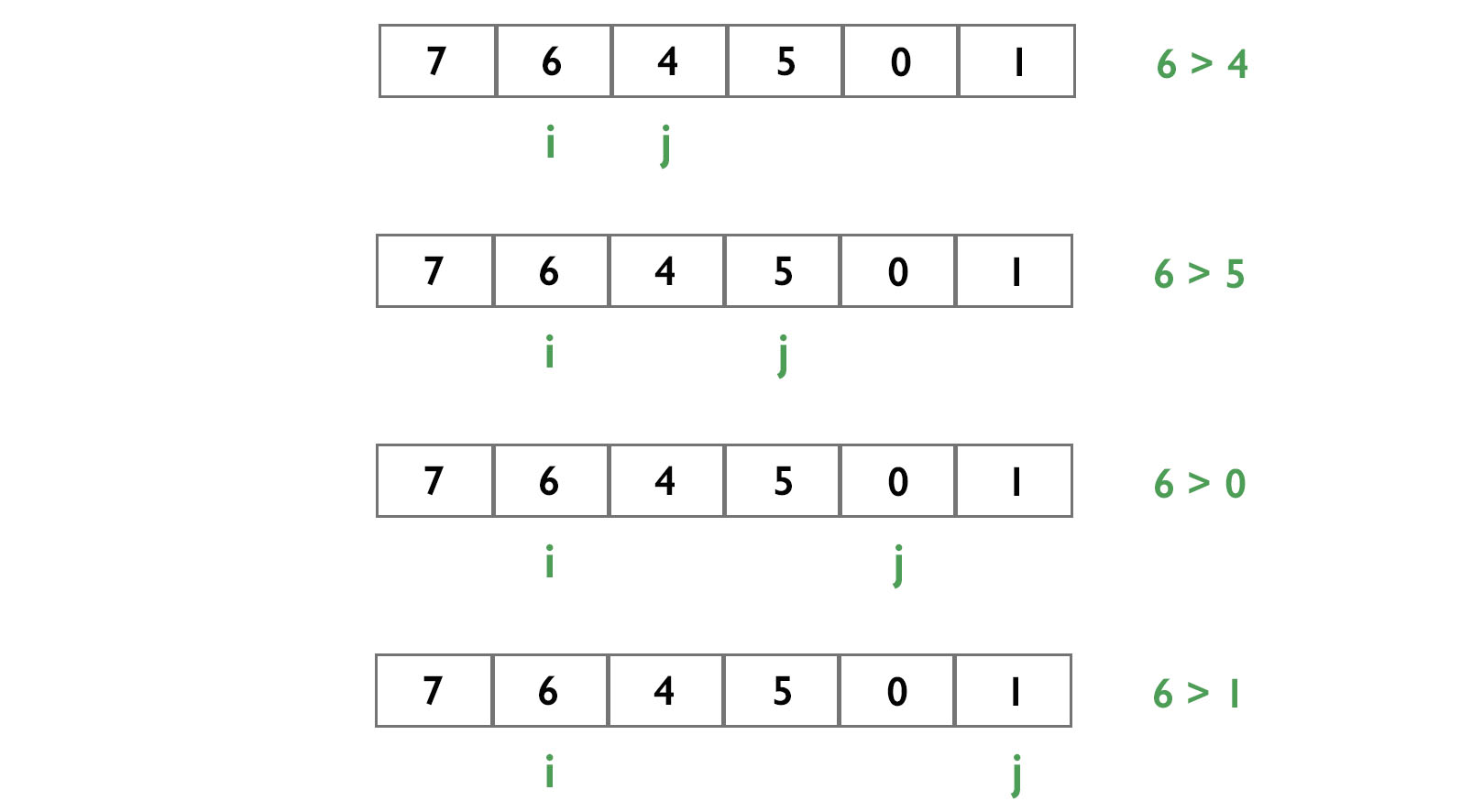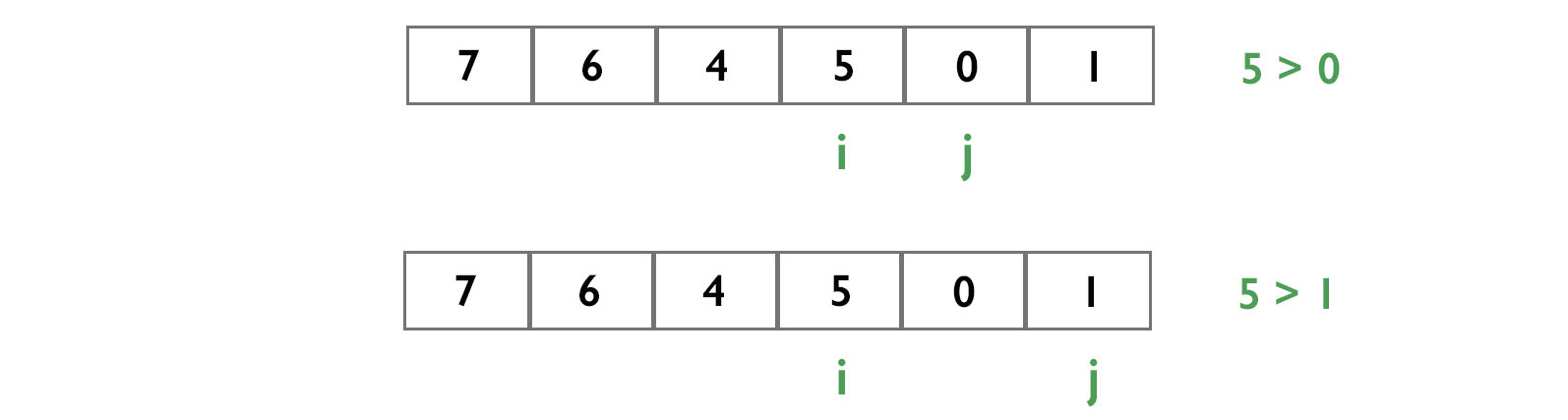July 5, 2020 15275

A leader in an array is an element which is greater than all the elements on its right side in the array.

Two types of elements are leaders by default.

1. Last element of an array is a leader by default because there is no element on the right side of it and so its right element is NULL.
2. Greatest element of an array is also a leader by default.

For example, take an array {2,5,8,7,3,6}. Here 6,7,8 are leaders. Let's take a look at them one by one.

6 - It is a leader by default because it is the last element of the array.

7 - It is a leader because it is greater than the elements on its right, i.e., 3,6.

8 - It is also a leader because it is greater than all the elements on its right, i.e., 7,3,6.

There are two methods for finding leaders in an array.

## Method 1

Run two loops. The outer loop runs from the left to the right of the array, in which i will point to the index of each element of the array. The inner loop runs from the i+1th position to the end of the array, in which j will point to the index of each element on the right side of the element picked by the outer loop. If the element picked by the outer loop is greater than all the elements on its right side picked by the inner loop, then the element is the leader.

Suppose our array is {7,6,4,5,0,1}.

In the first iteration of the outer loop, i will point to the index of the first element (7).Then we will make another pointer j which will point to the index of the i+1th element (6) and check if the jth element is greater than the ith element.If it is, then

-- We will break the loop and increment i by 1 and j will be (i+1).

else

-- We will increment j by 1.If the jth element is the last element, it means that none of the element to the right of the ith element is greater than the ith element, hence it(7) is a leader.

In the second iteration of the outer loop, i will point to the index of the second element (6).Hence, 6 is also a leader.

In the third iteration of the outer loop, i will point to the index of the third element (4).Here, 5 is greater than 4. This means that 4 is not a leader. Therefore, the loop will break and i will point to the index of the fourth element (5) and j to the index of the i+1th element (0).

In the fourth iteration of the outer loop, i will point to the index of the fourth element (5).Therefore, 5 is also a leader.

In the fifth iteration of the outer loop, i will point to the index of the fifth element (0).Since 0 is less than 1, 0 is not a leader. Therefore, the loop will break.

1 is also a leader because it is the last element of the array.

Therefore, in our example, 7, 6, 5 and 1 are leaders.

### Pseudo Code

for i=0 to n-1
for j = i to n-1
if array[i] < array[j]
break
if j == n-1
print array[i]

• C++
• Python
• Java
#include <stdio.h>

{
for (int i = 0; i < n; i++)
{
for (int j = i; j < n; j++)
{
if (arr[i] < arr[j])
{
break;
}

if (j == n - 1)
}
}
}

void main()
{
int arr[] = { 7, 6, 4, 5, 0, 1 };
int n = sizeof(arr) / sizeof(arr);

// calling function
}

# function for finding leaders

for i in range(0, n):
for j in range(i, n):
if (arr[i] < arr[j]):
break
if (j == n - 1):

arr = [ 7, 6, 4, 5, 0, 1 ]
n = len(arr)
# calling function

class Leaders
{
public static void leaderprint(int arr[], int n)
{
for (int i = 0; i < n; i++)
{
for (int j = i; j < n; j++)
{
if (arr[i] < arr[j])
{
break;
}

if (j == n - 1)
}
}
}

public static void main (String[] args)
{
int arr[] = { 7, 6, 4, 5, 0, 1 };
int n = arr.length;

// calling function
}
}


Output

7 is a leader


### Time Complexity

Since there are 2 for loops, time complexity is O(N x N).

## Method 2

Trick - Suppose we have to check if 8 is greater than {0,3,5}. Normally we will check if 8 is greater than 0/3/5 individually. We can also do it quickly. All we need to do is just find the maximum of {0,3,5} i.e. 5 and compare only 5 with 8.

We just need to check if an element of an array is greater than the maximum element among all the elements to its right.

We will make a variable. Let's name it leader (L) and assign it the last element of the array as it is a leader by default.

Let's understand this with an example.n is the size of the array i.e. 6

Here L is the last element ((n-1)th element) i.e. 1.

We will assign i to the index of the (n-2)th element (0) and will check if this element is greater than the leader (L). If it is, then

-- We will assign the ith element as the leader (L) and decrease i to (i-1)

else

-- We will decrease i to (i-1)

In our example, 0 is less than L i.e. 1. So, i will point to the index of 5.Now, 5 is greater than 1. So, 5 is also a leader and L will point to 5. i will point to the index of 4.This time, 4 is less than 5. So, i will point to the index of 6.Since 6 is greater than 5, 6 is also a leader and L will point to 6. i will point to the index of 7.7 is greater than 6. Hence 7 is also a leader.

### Pseudo Code

L = last element
print L

for i = n-2 to 0
if array[i] > L
L = array[i]
print L
• C++
• Python
• Java
#include <stdio.h>

{
// last element of an array is leader by default
int L = arr[n - 1];

// for finding leaders in other elements of the array
for (int i = n - 2; i >= 0; i--)
{
if (arr[i] > L)
{
L = arr[i];
}
}
}

void main()
{
int arr[] = { 7, 6, 4, 5, 0, 1 };
int n = sizeof(arr) / sizeof(arr);

}

# function for finding leaders

# last element of an array is leader by default
L = arr[n-1];

# for finding leaders in other elements of the array
for i in range(n-2, -1, -1):
if (arr[i] > L):
L = arr[i];

arr = [ 7, 6, 4, 5, 0, 1 ]
n = len(arr)
# calling function

class Ideone {

public static void leaderprint(int arr[], int n) {

// last element of an array is leader by default
int L = arr[n - 1];
System.out.println(L + " is a leader");

// for finding leaders in other elements of the array
for (int i = n - 2; i >= 0; i--)
{
if (arr[i] > L)
{
L = arr[i];
System.out.println(L + " is a leader");
}
}
}

public static void main(String[] args)
{
int[] arr = { 7, 6, 4, 5, 0, 1 };
int n = arr.length;

}
}


Output

1 is a leader